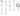# Count min sketch explained

Last time we looked at Bloom filters, which is one type of probabilistic data structure. In this post we will look at another one - Count Min Sketch.

In short, a count min sketch is used to:

• consume a stream of events, where each event has an event type
• query for the frequency of a particular event type in a sketch
• query for the frequency of a particular event type in two different sketches (this will give us the inner product of the frequencies of the event in the two sketches)

It's important to note that due to the risk of collisions and the size of the sketch, it can overestimate the true frequency of the events. It is after all a probabilistic data structure, it doesn't store the raw data, much like the Bloom filter, so this is expected.

So let's see how the sketch works.

We will need:

• a 2D array of `rows` x `rows` (`rows` and `cols` should be chosed based on the estimated number of unique event types inserted into the sketch)
• one hash function per row (the hash functions should be independent)

We'll start off with a blank 2D array.

``````// rows = 3, cols = 5
0 0 0 0 0
0 0 0 0 0
0 0 0 0 0``````

Now let's insert some events.

``````// This will insert an event of type "A".
insert("A")

// For each row, we'll hash the event type with the
// hashing function for that row to get an index k
// h0("A") - 2
// h1("A") - 4
// h2("A") - 0

// And now we index into every row with the computed k
// and increment by one.
0 0 1 0 0
0 0 0 0 1
1 0 0 0 0``````

Now an event of a different type.

``````insert("B")

// h0("B") - 1
// h1("B") - 4
// h2("B") - 3
0 1 1 0 0
0 0 0 0 2
1 0 0 1 0``````

Rememeber we are inserting a stream of events, so we will have many events of the same type. So let's insert another event of type "A";

``````insert("A")

// h0("A") - 2
// h1("A") - 4
// h2("A") - 0
0 1 2 0 0
0 0 0 0 3
2 0 0 1 0``````

Great, now how do we query for the frequency of an event?

``````getFrequency("A")

// This is our state
// 0 1 2 0 0
// 0 0 0 0 3
// 2 0 0 1 0

// We'll hash again, just like when inserting.
// h0("A") - 2
// h1("A") - 4
// h2("A") - 0

// Now for every row we'll get sketch[row, hrow("A")]
// sketch[0, 2] - 2 (the frequency on this row is 2)
// sketch[1, 4] - 3 (the frequency on this row is 3)
// sketch[2, 0] - 2 (the frequency on this row is 2)

// And now we take the min of those
// min(2, 3, 2)
2``````

Next up, we'll implement this. We already have all the building blocks for double hashing from the Bloom filter so the implementation should be pretty straight-forward.

``````void CountMinSketch::insert(const std::string & key)
{
for (size_t row = 0; row < m_rows; ++row)
{
// Hash
auto k = m_hash.hashIteration(key, row, m_cols);

// Increment
++(m_vector[row][k]);
}
}

uint32_t CountMinSketch::getFrequency(const std::string & key) const
{
size_t minFrequency = std::numeric_limits<std::size_t>::max();

for (size_t row = 0; row < m_rows; ++row)
{
// Hash
auto k = m_hash.hashIteration(key, row, m_cols);

// Find min
auto currentFrequency = m_vector[row][k];

if (currentFrequency < minFrequency)
{
minFrequency = currentFrequency;
}
}

return minFrequency;
}``````

You can see the full implementation at Sketch.